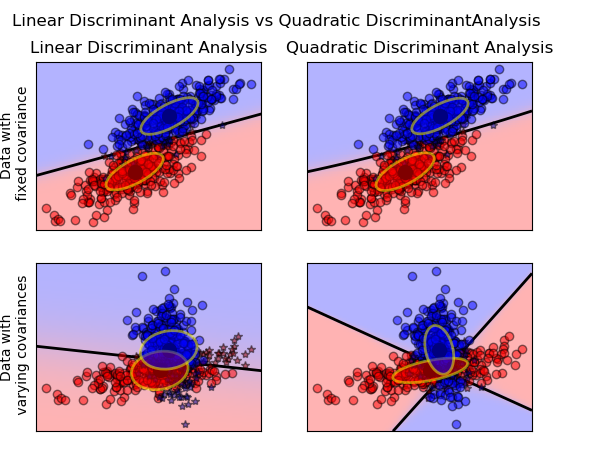### Linear Discriminant Analysis and Quadratic Discriminant Analysis.What is Linear Discriminant Analysis and Quadratic Discriminant Analysis?

Linear Discriminant Analysis or LDA is a statistical technique for binary and multiclass
classification. It too assumes a Gaussian distribution for the numerical input variables.

A quadratic classifier is used in machine learning and statistical classification to separate measurements of two or more classes of objects or events by a quadric surface. It is a more general version of the linear classifier.

Both are used dimension reduction to smaller but discriminative components.

Discriminant analysis is a composite procedure with two distinct stages – dimensionality reduction (supervised) and classification stage. At dimensionality reduction we extract discriminant functions which replace the original explanatory variables. Then we classify (typically by Bayes’ approach) observations to the classes using those functions.

Differences between LDA and QDA?

1. Linear Discriminant Analysis can only learn linear boundaries, while Quadratic Discriminant Analysis can learn quadratic boundaries and is therefore more flexible. Link

2. QDA can perform better in the presence of a limited number of training observations because it does make some assumptions about the form of the decision boundary.

3. QDA assumes a quadratic decision boundary, it can accurately model a wider range of problems than can the linear methods.

4. LDA is a much less flexible classifier than QDA.

References

http://scikit-learn.org/stable/modules/lda_qda.html

http://people.math.umass.edu/~anna/stat697F/Chapter4_2.pdf

https://stats.stackexchange.com/questions/169436/how-lda-a-classification-technique-also-serves-as-dimensionality-reduction-tec

Linear and Quadratic Discriminant Analysis for ML / statistics newbies